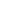### الصفحاتم4 الاهتزازات مرحلة رابعة

# تجارب مختبر الاهتزاز الميكانيكي Experiments of Mechanical Vibration

Experiments of

Mechanical

Vibration

Laboratory

نرفق لكم هذا الكتاب الالكتروني بصيغة pdf عبارة عن شيت لجميع تجارب المختبر كما مذكورة ادناة يغنيكم عن طلب الشيت من الاستاذ ويحتوي على جميع التجارب بالكامل مع الرسومات هذا الشيت مقدم من قبل مختبر الاهتزازات في جامعة البصرة كلية الهندسة قسم الميكانيك . بالتوفيق

Experiment No. 1 Simple Pendulum

Aim of the experiment

1. Validation of simple pendulum theory.

2. Estimation of gravitational acceleration, g.

=-=-=-=-=-=-=-=-=-==-=-=-=-=-=-=-=-=-=-=-=-=-=-=-=-=

Experiment No. 2 Mass-Spring Systems

Aim of the experiment

1. Verification of simple mass-spring system theory

2. Estimation of stiffness factor k for a spring

3. Estimation of gravitational acceleration g

=-=-=-=-=-=-=-=-=-==-=-=-=-=-=-=-=-=-=-=-=-=-=-=-=-=

Exp. No. 3 Torsional Vibration

Aim of the experiment

1. Estimation of the moment of inertia for a wheel.

2. Estimation of the damping coefficient.

=-=-=-=-=-=-=-=-=-==-=-=-=-=-=-=-=-=-=-=-=-=-=-=-=-=

Exp. No. 4 Forced Vibration with Negligible Damping

Aim of the Experiment:

1. Estimation of the natural frequency for a rigid body-spring system.

2. Verification of resonance condition.

=-=-=-=-=-=-=-=-=-==-=-=-=-=-=-=-=-=-=-=-=-=-=-=-=-=

Exp. No. 5 Two Degree of Freedom Torsional Vibration

Aim of the Experiment:

1. Estimation of the natural frequency for two rotor system.

2. Comparison of the theoretical and experimental frequencies.

=-=-=-=-=-=-=-=-=-==-=-=-=-=-=-=-=-=-=-=-=-=-=-=-=-=

Exp. No. 6 Whirling of Shafts

Aim of the Experiment:

1. Verification of Whirling theory.

2. Verification of Dunkerley's Equation.

=-=-=-=-=-=-=-=-=-==-=-=-=-=-=-=-=-=-=-=-=-=-=-=-=-=مهندس ميكانيك
هدفي هو التطوير من ذاتي ثم الاستفادة ثم تطوير غيري